CSDN

# 1.线性可分支持向量机

1.考虑一个线性二分类的问题；如下左图，在二维平面上有两种样本点x，目标值分别标记为{-1,1}，可以作出无数条直线wTx+b=0$w^Tx+b=0$,直线上方的点标记为{+1}的带入直线公式会得到wTx+b>0$w^Tx+b>0$,下方的点，标记为{-1}带入直线公式会得到wTx+b<0$w^Tx+b<0$，因此可以用wTx+b$w^Tx+b$的符号决定点的分类，写成决策函数为f(x,w,b)=sign(wTx+b)$f(x,w,b)=sign(w^Tx+b)$把两类点分开，但是个采用哪个直线最好呢？
2.一般来说，当样本点离直线越远，则分类正确的确信度越大；如下右图所示，A,B,C三个样本点都被预测分类到‘×’类中。但是对于A的分类正确的确信度比C大。因为点C里分类直线wTx+b=0$w^Tx+b=0$很近，当直线的斜率稍一点变化，即会导致C被分到另一类中。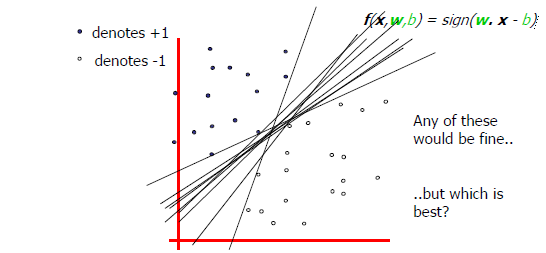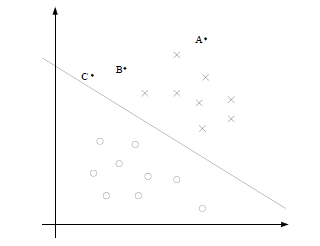## 1.1函数间隔与几何间隔

:γ^(i)=y(i)(wTx(i)+b)

γ^=mini=1,2,...m γ^(i)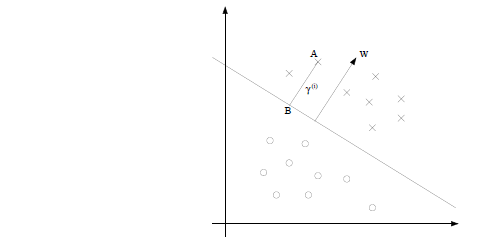wT(x(i)γ(i)w||w||)+b=0:γ(i)=(wTx(i)+b)||w||

:γ(i)=y(i)(wTx(i)+b||w||)

Sγ=mini=1,2,...m γ(i)

## 1.2间隔最大化

maxw,b γ

s.t. y(i)(wTx(i)+b||w||)γ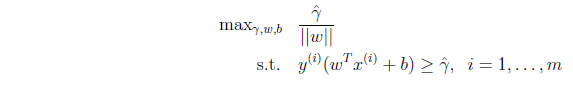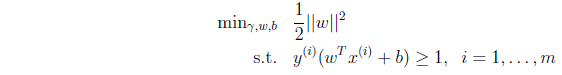## 1.3拉格朗日对偶性

f(w),gi(w),hi(w)$f(w),g_i(w),h_i(w)$均为连续可微：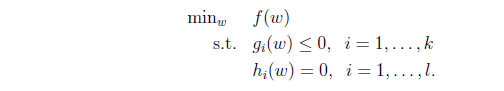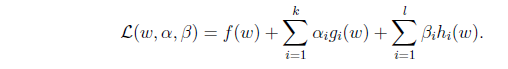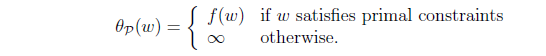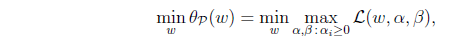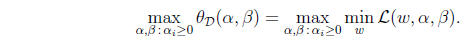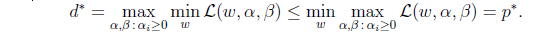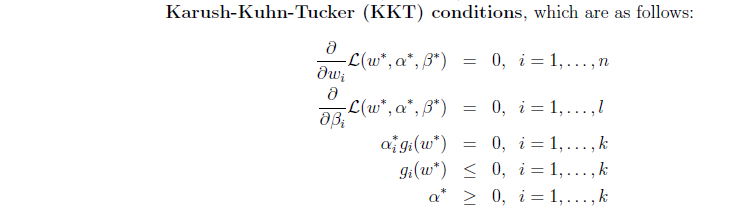## 1.4最优间隔分类器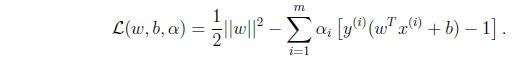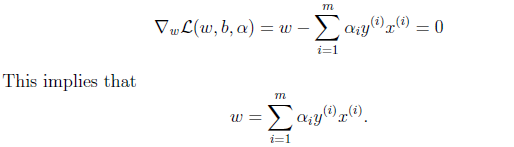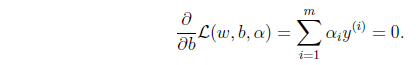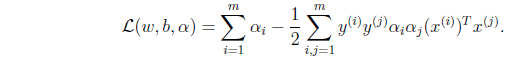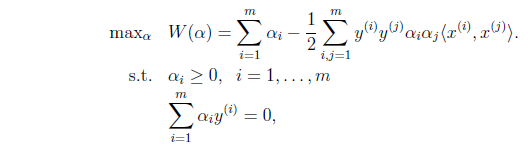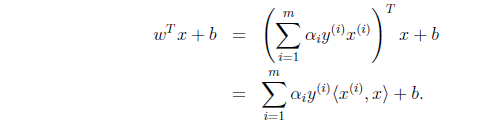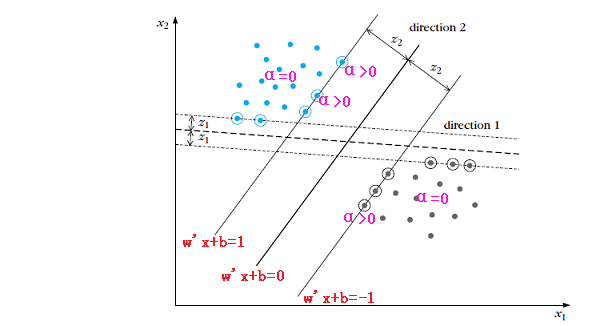# 2.线性支持向量机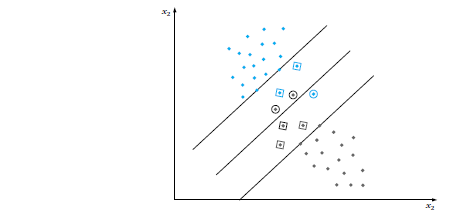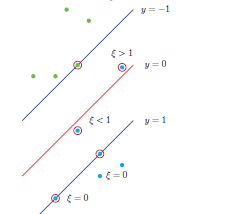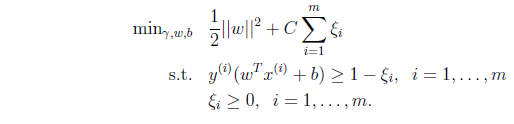C称为惩罚参数(C>0)，C值越大对误分类的惩罚越大；因为当C为无穷大时，即成为了线性可分问题。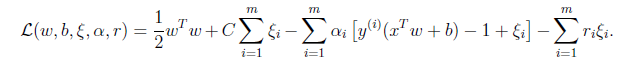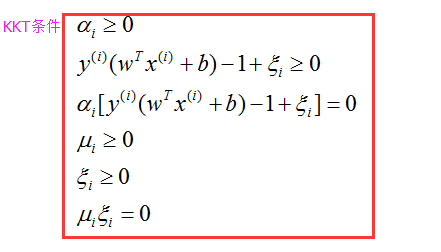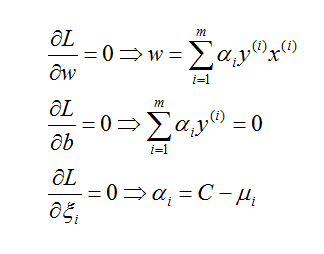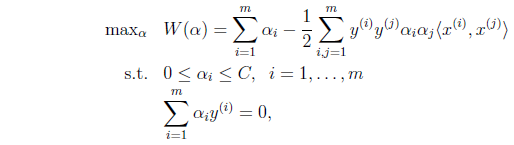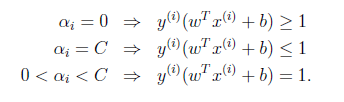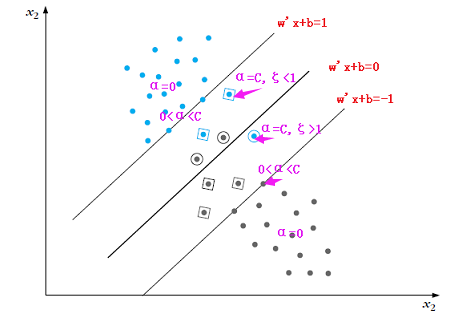# 3.非线性支持向量机

## 3.1 概念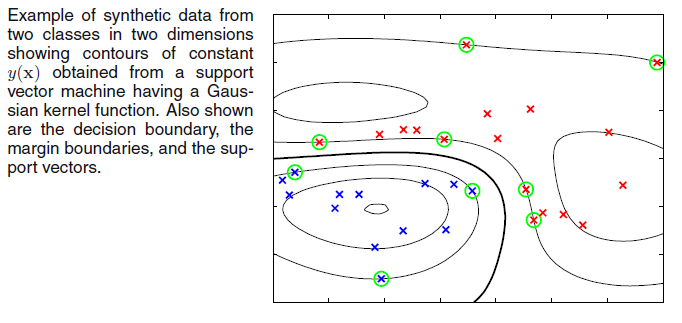## 3.2 核技巧Eg:x,zRn, K(x,z)=(xTz)2=i,j=1n(xixj)(zizj),ϕ(x)=(x1x1,x1x2...,x1xn,x2x1,...xnxn)T

K(x,z)=(xTz+1)q,q>0

K(x,z)=exp(||xz||22σ2),

K(x,z)=tanh(βxTz+γ)Mercerβ=2,γ=1

# 4.SVM后验概率输出

SVM分类器中判决函数y^=sign(f(x))=sign(wTx+b)$\hat{y}=sign(f(x))=sign(w^Tx+b)$。可以采用f(x)与sigmoid函数结合，把f(x)=wTx+b$f(x)=w^Tx+b$解释成y=1$y=1$的对数几率,SVM分类器概率输出(Platt 2000)：

p(y^=1|x)=σ(Af(x)+B)

# 5.几种损失函数的比较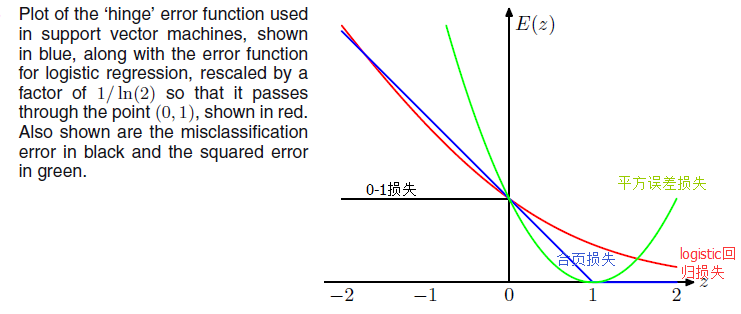## 5.1合页损失函数

minw,b i=1m[1y(i)(wTx(i)+b)]++λ||w||2

λ=12C12||w||2+Ci=1mξi

## 5.2logistic回归损失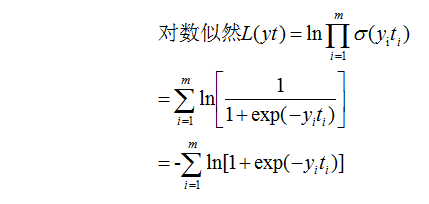i=1mln[1+exp(yiti)]+λ||w||2

## 5.3最小二乘损失

i=1m(yiti)2+λ||w||2

# 6.SVM多分类问题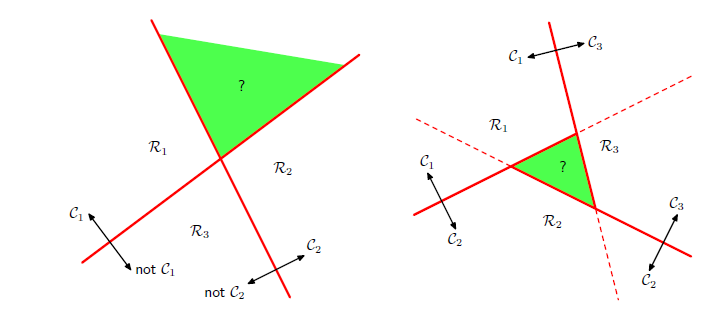## 3.其他方法

1.一次训练K分类的SVM。参数多，复杂度高；(Weston and Watkins 1999)
2.DAGSVM ;(Platt 2000)

1. PRML
2. MLAPP
3. CS 229-Andrew Ng©️2019 CSDN 皮肤主题: 大白 设计师: CSDN官方博客